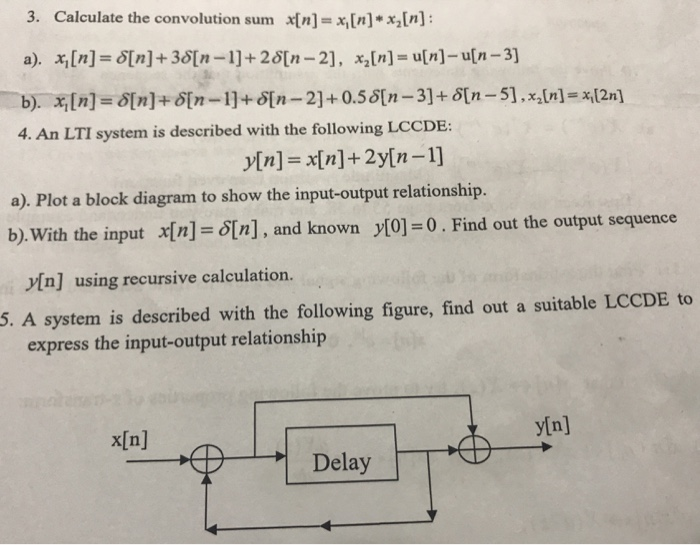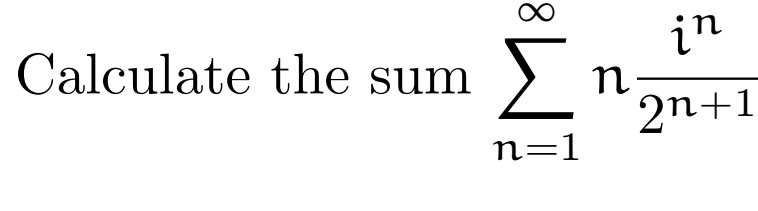Question

# How to calculate this sum? S=(x+1/x)^2+(x^2+1/(x^2))^2+...+(x^n+1/(x^n))^2.

How to calculate this sum? S=(x+1/x)^2+(x^2+1/(x^2))^2+...+(x^n+1/(x^n))^2.

Answer 1

$S = {\left(x + \frac{1}{x}\right)}^{2} + {\left({x}^{2} + \frac{1}{{x}^{2}}\right)}^{2} + \ldots + {\left({x}^{n} + \frac{1}{{x}^{n}}\right)}^{2}$

$S = \left({x}^{2} + {x}^{4} + {x}^{6} + \ldots + {x}^{2 n}\right) + \left(\frac{1}{x} ^ 2 + \frac{1}{x} ^ 4 + \frac{1}{x} ^ 6 + \ldots + \frac{1}{x} ^ \left(2 n\right)\right) + 2 n$

$S = \frac{{x}^{2} \left({x}^{2 n} - 1\right)}{{x}^{2} - 1} + \left(\frac{\frac{1}{x} ^ 2 \left(1 - \frac{1}{x} ^ \left(2 n\right)\right)}{1 - \frac{1}{x} ^ 2}\right) + 2 n$

Taking $x > 1$

#### Earn Coins

Coins can be redeemed for fabulous gifts.

Similar Homework Help Questions

• ### Calculate the convolution sum x{n]=x[n]*x,[n]: 3. a). xn] S[n]+36[n-1]+28[n-2], x,[n]- u[n]- u[n-3) b). [n]- S[n]+ d[n=1]+S[n-2]+0.58[n-3]+...Calculate the convolution sum x{n]=x[n]*x,[n]: 3. a). xn] S[n]+36[n-1]+28[n-2], x,[n]- u[n]- u[n-3) b). [n]- S[n]+ d[n=1]+S[n-2]+0.58[n-3]+ S[n-51,x,[n]- x,[2n] 4. An LTI system is described with the following LCCDE: In]=x[n]+2y[n-1] a). Plot a block diagram to show the input-output relationship. b).With the input x[n]= S[n], and known y = 0 . Find out the output sequence In] using recursive calculation. 5. A system is described with the following figure, find out a suitable LCCDE to express the input-output relationship y[n] [n]...

• ### Calculate the sum 〉 n 2n+1 Calculate the sum 〉 n 2n+1Calculate the sum 〉 n 2n+1 Calculate the sum 〉 n 2n+1